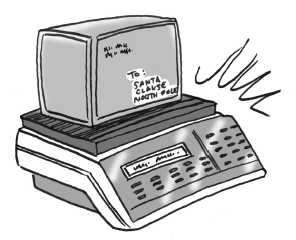### Home > CCA > Chapter 3 > Lesson 3.2.3 > Problem3-61

3-61.Mailboxes Plus sends packages overnight for $\5$ plus $\0.25$ per ounce. United Packages charges $\2$ plus $\0.35$ per ounce. Mr. Molinari noticed that his package would cost the same to mail using either service. How much does his package weigh?

Write equations for both brands.

$y=$ total cost
$x=$ weight in ounces

Set the equations equal to each other to solve for $x$.